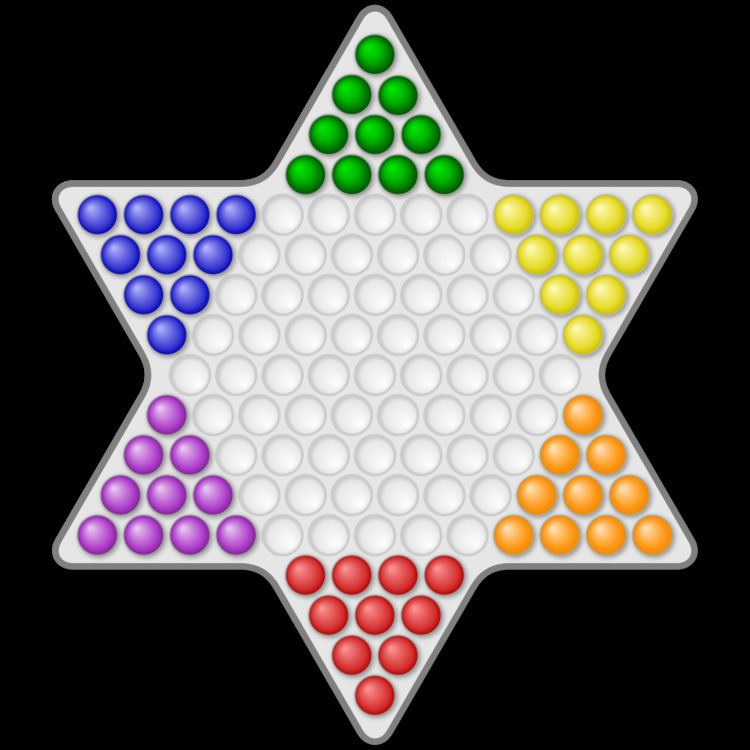# 121 (number)

Updated on
Covid-19
 121  122 →Factorization  11Roman numeral  CXXI Cardinal  one hundred twenty-oneDivisors  1, 11, 121 121Ordinal  121st (one hundred and twenty-first)

121 (one hundred [and] twenty-one) is the natural number following 120 and preceding 122.

## In mathematics

One hundred [and] twenty-one is a square and is the sum of three consecutive primes (37 + 41 + 43). There are no squares besides 121 known to be of the form 1 + p + p 2 + p 3 + p 4 , where p is prime (3, in this case). Other such squares must have at least 35 digits.

There are only two other squares known to be of the form n! + 1, supporting Brocard's conjecture. Another example of 121 being of the few examples supporting a conjecture is that Fermat conjectured that 4 and 121 are the only perfect squares of the form x3 - 4 (with x being 2 and 5, respectively).

It is also a star number and a centered octagonal number.

In base 10, it is a Smith number since its digits add up to the same value as its factorization (which uses the same digits) and as a consequence of that it is a Friedman number (11^2). But it can not be expressed as the sum of any other number plus that number's digits, making 121 a self number.

## In other fields

121 is also:

• The electricity emergency telephone number in Egypt
• The number for voicemail for mobile phones on the Vodafone network
• The undiscovered chemical element Unbiunium has the atomic number 121
• The official end score for Cribbage
• ## References

Similar Topics
Red Cliff (film)
Cody Asche
Shehla Raza
Topics

 B i Link H2
 L